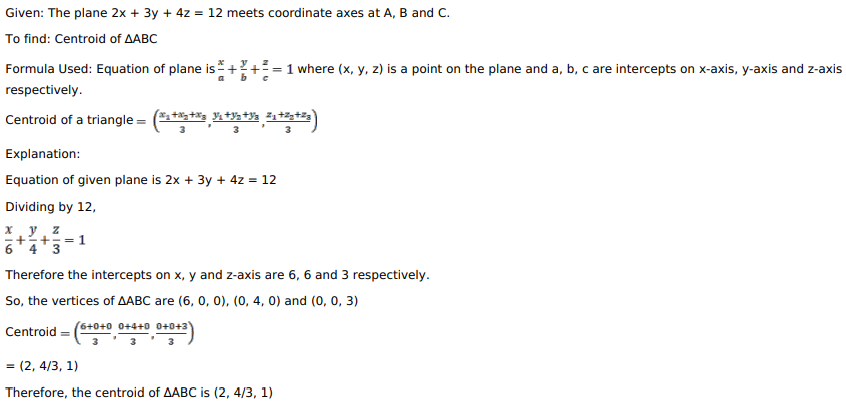# Mark against the correct answer in each of the following:Question:

Mark against the correct answer in each of the following:

The plane $2 x+3 y+4 z=12$ meets the coordinate axes in $A, B$ and $C$. The centroid of $\triangle A B C$ is

A. $(2,3,4)$

B. $(6,4,3)$

C. $\left(2, \frac{4}{3}, 1\right)$

D. None of these

Solution: